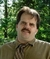## Auto populating cells in Excel based on timeTags:
Microsoft Excel
Visual Basic
So I am given times of the day and I need to match the shift with the time so I was wondering if there was a macro that would make this easy. For example, between 07:00 and 15:00 is shift 1 and 15:00 to 23:00 is shift two and the other period is shift 3. I'm sure that there is a loop for this but it needs to continue until there are no more excel lines on the sheet (usually about 3500 lines). If anyone could point in the right direction on how to orchestrate this loop, it would be very much appreciated.

Software/Hardware used:
excel, visual basic

Thanks. We'll let you know when a new response is added.

## Discuss This Question: 2 Replies

Thanks. We'll let you know when a new response is added.
•Post the code you have tried and we can take a look at your logic. It's probably a minor tweak.
report
• Dim sht As Worksheet
Dim LastRow As Long
Dim LastColumn As Long
Dim StartCell As Range
Dim Shift As Integer
Dim time As Date

' Select cell H2, *first line of data*.
Range("H2").Select
' Set Do loop to stop when an empty cell is reached.
Do Until IsEmpty(ActiveCell)

If 0.6256018519 <= Int(Cells(2, 6000).Value) > 0.2916782407 Then

Cells(2, 6000) = 1

ElseIf 0.958333333333 <= Int(Cells(2, 6000).Value) > 0.6256018519 Then

Cells(2, 6000) = 2

Else

Cells(2, 6000) = 3

End If
' Insert code here.
' Step down 1 row from present location.
ActiveCell.Offset(1, 0).Select
Loop

End Sub

' I know it is pretty sloppy right now but I feel like I've tried several different ways and just cant seem to get it working. Any guidance would help so much.
report